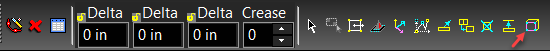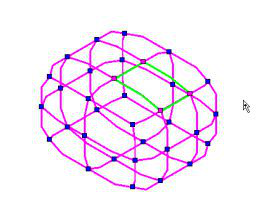Sometimes it is desirable to increase the number of faces in a given area. This is called refining and can be done to any selected face. You cannot refine if the Edit tool is in Selector mode, or if the Smoothness Level of the object is set to none. To refine a face or set of faces, first select them with the Edit tool. Then select the Refine Face option from the local menu or from the Inspector bar.The face will be refined based on the Smoothness Level. In essence the invisible subdivisions are converted into new faces for the object. The degree of refinement is as follows: If the Smoothness Level = 1 the then face (with four edges) will be divided into 2 x 2 (4 new faces) If the Smoothness Level = 2 the then face (with four edges) will be divided into 4 x 4 (16 new faces) If the Smoothness Level = 3 the then face (with four edges) will be divided into 8 x 8 (64 new faces) If the Smoothness Level = 4 the then face (with four edges) will be divided into 16 x 16 (256 new faces) At a minimum, one new face will be made for each edge of the face. The general rule of thumb for the algorithm is: Where F is final number of faces, N is the Number of initial edges, S is the Smoothness Level. F=N(2^S) So if the smoothness Level is 3, and the number of edges is 6, the resulting number of faces will be 96.